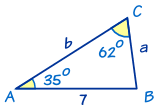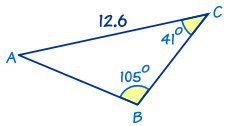# Solving AAS Triangles

"AAS" means "Angle, Angle, Side""AAS" is when we know two angles and one side (which is not between the angles).

To solve an AAS triangle

### Example 1In this triangle we know:

• angle A = 35°
• angle C = 62°
• and side c = 7

We can first find angle B by using 'angles of a triangle add to 180°':

B = 180° − 35° − 62° = 83°

To find side a we can use The Law of Sines:

a/sin(A) = c/sin(C)

a/sin(35°) = 7/sin(62°)

Multiply both sides by sin(35°):

a = sin(35°) × 7/sin(62°)
a = 4.55 to 2 decimal places

To find side b we can also use The Law of Sines:

b/sin(B) = c/sin(C)

b/sin(83°) = 7/sin(62°)

Multiply both sides by sin(83°):

b = sin(83°) × 7/sin(62°)
b = 7.87 to 2 decimal places

Now we have completely solved the triangle!

We used b/sin(B) = c/sin(C) rather than b/sin(B) = a/sin(A) for the last calculation ... why?

There's a good reason for that. What if we made a mistake when finding a? Then our answer for b would also be wrong!

As a rule, it is always better to use the sides and angles that are given rather than ones we've just worked out.

### Example 2This is also an AAS triangle.

First find angle A by using "angles of a triangle add to 180°":

A = 180° − 41° − 105° = 34°

Now find side c by using The Law of Sines:

c/sin(C) = b/sin(B)
c/sin(41°) = 12.6/sin(105°)
c = sin(41°) × 12.6/sin(105°)
c = 8.56 to 2 decimal places

Similarly we can find side a by using The Law of Sines and using the given side b = 12.6 (rather than side c that we just worked out):

a/sin(A) = b/sin(B)
a/sin(34°) = 12.6/sin(105°)
a = sin(34°) × 12.6/sin(105°)
a = 7.29 to 2 decimal places

Done!

261, 3956, 1540, 262, 1541, 1542, 1560, 2370, 2371, 3955